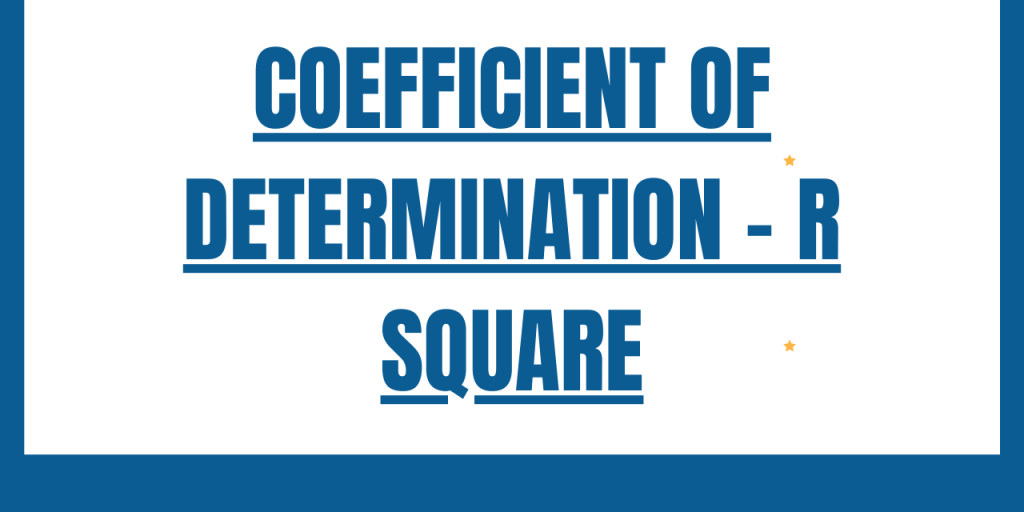# Coefficient of Determination – R squared value in PythonHello, readers! In this article, we will be focusing on the Coefficient of Determination in Python. So, let us get started! 🙂

## What is the Coefficient of Determination (R squared value)?

Before diving deep into the concept of Coefficient of Determination, let us first understand the necessity of evaluation of a machine learning model through error metrics.

In the domain of Data Science, to solve any model it is very necessary for the engineer/developer to evaluate the efficiency of a model prior to applying it to the dataset. The evaluation of the model is based on certain error metrics. The coefficient of determination is one such error metric.

Coefficient of Determination also popularly known as R square value is a `regression error metric` to evaluate the accuracy and efficiency of a model on the data values that it would be applied to.

R square values describe the performance of the model. It describes the variation in the response or target variable which is predicted by the independent variables of the data model.

Thus, in simple words we can say that, the R square value helps determine how well the model is blend and how well the output value is explained by the determining(independent) variables of the dataset.

The value of R square ranges between [0,1]. Have a look at the below formula!

R2= 1- SSres / SStot

Here,

• SSres represents the sum of squares of the residual errors of the data model.
• SStot represents the total sum of the errors.

Higher is the R square value, better is the model and the results.

## R square with NumPy library

Let us now try to implement R square using Python NumPy library.

We follow the below steps to get the value of R square using the Numpy module:

1. Calculate the Correlation matrix using `numpy.corrcoef()` function.
2. Slice the matrix with indexes [0,1] to fetch the value of R i.e. `Coefficient of Correlation`.
3. Square the value of R to get the value of R square.

Example:

```import numpy
actual = [1,2,3,4,5]
predict = [1,2.5,3,4.9,4.9]

corr_matrix = numpy.corrcoef(actual, predict)
corr = corr_matrix[0,1]
R_sq = corr**2

print(R_sq)

```

Output:

```0.934602946460654
```

## R square with Python sklearn library

Now, let us try to calculate the value of R square using sklearn library. Python sklearn library provides us with an r2_score() function to determine the value of the coefficient of determination.

Example:

```from sklearn.metrics import r2_score
a =[1, 2, 3, 4, 5]
b =[1, 2.5, 3, 4.9, 5.1]
R_square = r2_score(a, b)
print('Coefficient of Determination', R_square)

```

Output:

```Coefficient of Determination 0.8929999999999999
```

## Conclusion

By this, we have come to the end of this topic. Feel free to comment below, in case you come across any question. For more such posts related to Python, Stay tuned and till then. Happy Learning!! 🙂